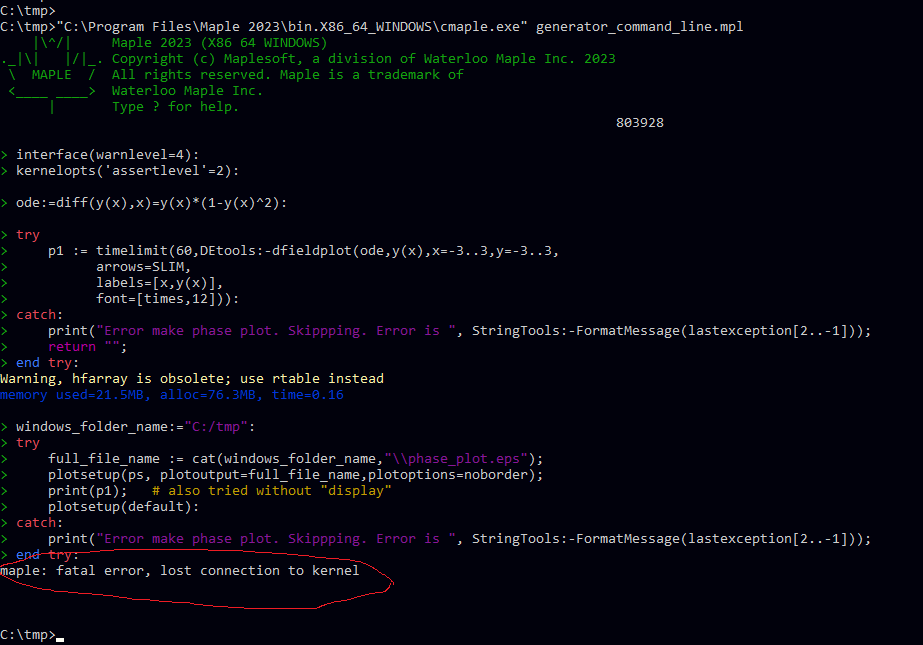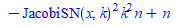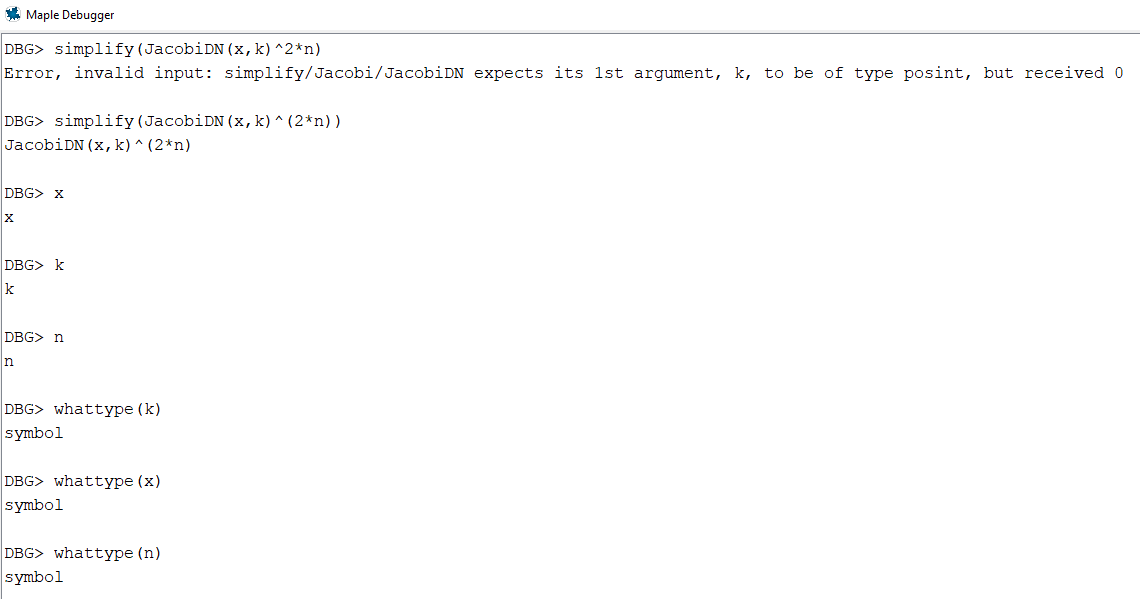## maple: fatal error, lost connection to kernel when...

Please ignore. Just found the problem googling around an old question.

I needed to REMOVE font=[times,12]. Now it works.

I tried first to change it to font=[TIMES,12] and thought it worked, but it did not. So the solution is to simply remove the font settings!

And now the crash is gone. At least for this one case. I need to do more testing for other cases. But this seems to be the problem. It was the font settings.

----------------------------------------- closed/resolved ------------------------------------------

I have been trying to move my my test mainline from the worksheet to .mpl and to run the .mpl from the command line usuing cmaple.exe hoping that the slow down due to large output into the worksheet will be elminated.

This is all on windows. So I am using DOS/Command line.

Everthing seems to be working fine, and it seems to run faster now, but I get maple: fatal error, lost connection to kernel on some problems.

After some debugging I found where it happens. In the code, I generates plots and then save each to .eps file.  This all works find when I run the code from the worksheet ofcourse.

Below I show an example .mpl and the command I used. I also show same code works just fine in the worksheet.

I suspect when running cmaple.exe it is using different driver for postscript than the one used when running maple.exe?  If so, what to change in the code to make it work OK from command line?

This is the .mpl file. You can put this anywhere. Say C:/tmp/ folder. First this is screen shot of the errorHere is the .mpl file

```interface(warnlevel=4):
kernelopts('assertlevel'=2):

ode:=diff(y(x),x)=y(x)*(1-y(x)^2):

try
p1 := timelimit(60,DEtools:-dfieldplot(ode,y(x),x=-3..3,y=-3..3,
arrows=SLIM,
labels=[x,y(x)],
font=[times,12])):
catch:
print("Error make phase plot. Skippping. Error is ", StringTools:-FormatMessage(lastexception[2..-1]));
return "";
end try:

try
full_file_name := "C:\\tmp\\phase_plot.eps": #same problem using .ps extension
plotsetup(ps, plotoutput=full_file_name,plotoptions=noborder);
print(p1);   # also tried without "display"
plotsetup(default):
catch:
print("Error make phase plot. Skippping. Error is ", StringTools:-FormatMessage(lastexception[2..-1]));
end try:```

Here is the command used

`"C:\Program Files\Maple 2023\bin.X86_64_WINDOWS\cmaple.exe" generator_command_line.mpl`

The crash happens even if I remove the kernelopts('assertlevel'=2):

To reproduce, simply put the above .mpl file into your c:\tmp folder and start the windows command line (DOS) and type the above command.

Important: if I use different device, say "gif" instead of "ps", then Maple does not crash. But I need to use "ps" in order to convert to pdf as I need vector graphics which is much better quality. So it looks like the ps driver used by cmaple.exe is the problem, but I am not sure. But looking for a workaround as without the ability to generate the plots, this setup will not work for me.

I rememebr now seeing this problem many years ago, but never was able to find workaround.

Any workaround I should try? I need to try the command line, hoping the frequent hangs and slow down will go away if I do that.

Maple 2023 on windows 10.

## Annotation in plots...

I am trying to put an annotation  (dimension of a distance) into a plot.

Any hints about what would the best way to do that?## Problem in executing the solve command???...

hi,

Does anyone know why my code is not running?

## bad hang in call to int(). Will not time out using...

My program simply keeps hanging.

It takes me 5 days to complete something which should take 2-3 hrs, since Maple keeps hanging, and I have to keep terminating server.exe and start again. Sometimes when I start again it does not hang where it was. I do this dozens of time per day.

even though I use timelimit on every possible Maple call. The problem also is that when timelimit works, it takes 10-20 times more time than asked. I ask for 10 second timeout, sometimes it timesout after 3-10 minutes if I am lucky.

So I keep trying to make workarounds and I am tired of all of this. Just waste of time. This is getting worst with each new Maple release not better.

Here is an example

```restart;
expr:=-1/3*2^(2/3)/((a^2*p+2*a*p^2+p^3+4)/p)^(1/2)*((-1/2*a^2*p-1/2*p^2*a+1/2*p*((a^2*p+2*a*p^2+p^3+4)/p)^(1/2)*a-3)*(-p^2*(-((a^2*p+2*a*p^2+p^3+4)/p)^(1/2)+a+p))^(2/3)+2^(2/3)*(-(a+3/2*p)*p*((a^2*p+2*a*p^2+p^3+4)/p)^(1/2)+a^2*p+5/2*p^2*a+3/2*p^3+3)*p)/(-((a^2*p+2*a*p^2+p^3+4)/p)^(1/2)+a+p)/(-1/2*2^(2/3)*(-p^2*(-((a^2*p+2*a*p^2+p^3+4)/p)^(1/2)+a+p))^(2/3)+p*(p*(-p^2*(-((a^2*p+2*a*p^2+p^3+4)/p)^(1/2)+a+p))^(1/3)+2^(1/3)))/p^2;

try
t0:=time[real]();
timelimit(20,int(expr,p));
catch:
print("time used ",time[real]()-t0,"seconds");
print("timed out");
end try;
```

in Maple 2023, with 128 GB RAM and very fast PC and nothing else is running, it hangs. I could leave it for hrs, the server.exe  is running at full CPU and timelimit is ignored. Timelimit in Maple is useless.

I do not know what else to do. if someone can suggest something, I am willing to try anything before I finally give up.## 2d plot error for ode solve by FDM...

Hello

I have implemented FDM for  nonlinear coupled equation

I that I have to plot U versus Y=i*h and C versus Y

As well Theta(T) versus Y

And I want to calculate U(0.5) T(0.5) and C(0.5)

For different values of M

Like M=1,2,3

Please give me command for the plot and table value of U ,T,&C (0.5)

 > restart; # Parameter values:  Pr:=1:E:=1:A:=0.1:Sc:=0.01: K:=0.5: a := 0: b := 1: N := 9: h := (b-a)/(N+1): k := (b-a)/(N+1):  lambda:= 1/h^2:  lambda1:= 1/k^2: # Initial conditions for i from 0 to N do   U[i, 0] := h*i+1: end do: for i from 0 to N do   T[i, 0] := h*i+1: end do: for i from 0 to N do   C[i, 0] := h*i+1: end do: # Boundary conditions for j from 0 to N+1 do   U[0, j] := exp(A*j*lambda);   U[N+1, j] := 0;   T[0, j] := j*lambda1;   T[N+1, j] := 0;   C[0, j] := j*lambda1;   C[N+1, j] := 0 end do: #Discretization Scheme for i to N do   for j from 0 to N do     eq1[i, j]:= lambda1*(U[i, j+1]-U[i, j]) = (Gr/2)*(T[i, j+1]+T[i,j])+(Gr/2)*(C[i, j+1]+C[i,j])+(lambda^2/2)*(U[i-1,j+1]-2*U[i,j+1]+U[i+1,j+1]+U[i-1,j]-2*U[i,j]+U[i+1,j])-(M/2)*(U[i,j+1]+U[i,j]) ;     eq2[i, j]:= lambda1*(T[i, j+1]-T[i, j]) = (1/Pr)*(lambda^2/2)*(T[i,j+1]-2*T[i,j+1]+T[i+1,j+1]+T[i-1,j]-2*T[i,j]+T[i+1,j])+(E*lambda^2)*((U[i+1,j]-U[i,j])^2);     eq3[i, j]:= lambda1*(C[i, j+1]-C[i, j]) = (1/Sc)*(lambda^2/2)*(C[i,j+1]-2*C[i,j+1]+C[i+1,j+1]+C[i-1,j]-2*C[i,j]+C[i+1,j])+(K/2)*((C[i,j+1]+C[i,j]))     end do end do:
 > `union`(  seq(seq( indets( eq1[i,j], name), i=1..N), j=0..N),             seq(seq( indets( eq2[i,j], name), i=1..N), j=0..N),             seq(seq( indets( eq3[i,j], name), i=1..N), j=0..N)           );    numelems(%):   numelems(eq1)+numelems(eq2)+numelems(eq3);(1)
 > fsolve( eval( [ seq(seq(eq1[i,j], i=1..N),j=0..N),                   seq(seq(eq2[i,j], i=1..N),j=0..N),                   seq(seq(eq3[i,j], i=1..N),j=0..N)                 ],                 [Gr=1.0, M=2]               )         );
 > plot(eval([seq([i*h, U])],thickness = 4, axes = boxed, labels = [U, y], color = red, title = "FDM"));
 >## Error, (in Algebraic:-MakeMonic) assertion failed ...

I do not know if this is known issue. In V 2023  I was calling odetest on solution to ode. It gives the above internal exception.

This happens when there is signum's in the solution and shows up when kernelopts('assertlevel'=2): is set.

Code is below. Could someone verify if they see this or not and if this looks like a new bug? If so, will send email to support.

When I replaced all the signum(...) calls by 1, the exception went away. I do not know now if this happens in V 2022 or not.

The problem with these internal exception is that they can not be cought. So the whole program crash even when using try/catch.

```restart;
interface(warnlevel=4);
kernelopts('assertlevel'=2):
ode:=3*x^2+9*y(x)*x+5*y(x)^2-(6*x^2+4*y(x)*x)*diff(y(x),x) = 0;

current_sol_for_y:=x = -1805/9*(x^2+y(x)*x+1/3*y(x)^2)^2*(7/8*((x+10/7*y(x))*abs(x)+10/21*signum(x)*y(x)^2)*signum(3*x+2*y(x))*signum(21*x^2+30*y(x)*x+10*y(x)^2)+x^2+5/4*y(x)*x+5/12*y(x)^2)^2*(x^2+25/19*y(x)*x+25/57*y(x)^2)^2*c/(11/9*(21/44*abs(x)^3+y(x)*(x+15/22*y(x))*abs(x)+5/33*signum(x)*y(x)^3)*signum(3*x+2*y(x))*signum(21*x^2+30*y(x)*x+10*y(x)^2)+(x^2+5/4*y(x)*x+5/12*y(x)^2)*(x+2/3*y(x)))^2/x^4/(x+2/3*y(x))^2;

odetest(current_sol_for_y,ode)

```

 > interface(version);> restart;> interface(warnlevel=4); kernelopts('assertlevel'=2):> ode:=3*x^2+9*y(x)*x+5*y(x)^2-(6*x^2+4*y(x)*x)*diff(y(x),x) = 0;> current_sol_for_y:=x = -1805/9*(x^2+y(x)*x+1/3*y(x)^2)^2*(7/8*((x+10/7*y(x))*abs(x)+10/21*signum(x)*y(x)^2)*signum(3*x+2*y(x))*signum(21*x^2+30*y(x)*x+10*y(x)^2)+x^2+5/4*y(x)*x+5/12*y(x)^2)^2*(x^2+25/19*y(x)*x+25/57*y(x)^2)^2*c/(11/9*(21/44*abs(x)^3+y(x)*(x+15/22*y(x))*abs(x)+5/33*signum(x)*y(x)^3)*signum(3*x+2*y(x))*signum(21*x^2+30*y(x)*x+10*y(x)^2)+(x^2+5/4*y(x)*x+5/12*y(x)^2)*(x+2/3*y(x)))^2/x^4/(x+2/3*y(x))^2:
 > odetest(current_sol_for_y,ode)

Error, (in Algebraic:-MakeMonic) assertion failed in assignment to p, expected algfun, got (312500*signum(x)^2*_X000001+625000*signum(x)*_X000001+312500*_X000001)*_Z^12+(3750000*x*signum(x)^2*_X000001+9375000*x*signum(x)*_X000001+1875000*abs(x)*signum(x)*_X000001+5625000*x*_X000001+1875000*abs(x)*_X000001)*_Z^11+(20175000*x^2*signum(x)^2*_X000001+64350000*x^2*signum(x)*_X000001+23812500*x*abs(x)*signum(x)*_X000001+46987500*x^2*_X000001+29437500*x*abs(x)*_X000001+2812500*abs(x)^2*_X000001)*_Z^10+(63450000*x^3*signum(x)^2*_X000001+265950000*x^3*signum(x)*_X000001+136800000*x^2*abs(x)*signum(x)*_X000001+240750000*x^3*_X000001+212737500*x^2*abs(x)*_X000001+37687500*x*abs(x)^2*_X000001)*_Z^9+(6400*x^5*signum(x)^2+127399500*x^4*signum(x)^2*_X000001+19200*x^5*signum(x)+732339000*x^4*signum(x)*_X000001+465435000*x^3*abs(x)*signum(x)*_X000001+14400*x^5+842314500*x^4*_X000001+932985000*x^3*abs(x)*_X000001+230203125*x^2*abs(x)^2*_X000001)*_Z^8+(19200*x^6*signum(x)^2+167022000*x^5*signum(x)^2*_X000001+144000*x^6*signum(x)+57600*x^5*abs(x)*signum(x)+1403001000*x^5*signum(x)*_X000001+1030887000*x^4*abs(x)*signum(x)*_X000001+172800*x^6+86400*x^5*abs(x)+2119149000*x^5*_X000001+2755512000*x^4*abs(x)*_X000001+841792500*x^3*abs(x)^2*_X000001)*_Z^7+(14400*x^7*signum(x)^2+139455000*x^6*signum(x)^2*_X000001+434880*x^7*signum(x)+257280*x^6*abs(x)*signum(x)+1892559600*x^6*signum(x)*_X000001+1537209900*x^5*abs(x)*signum(x)*_X000001+911520*x^7+774720*x^6*abs(x)+3929588100*x^6*_X000001+129600*x^5*abs(x)^2+5746914900*x^5*abs(x)*_X000001+2035037250*x^4*abs(x)^2*_X000001)*_Z^6+(67716000*x^7*signum(x)^2*_X000001+660960*x^8*signum(x)+383040*x^7*abs(x)*signum(x)+1773867600*x^7*signum(x)*_X000001+1538222400*x^6*abs(x)*signum(x)*_X000001+40320*x^5*abs(x)^3*signum(x)+2760480*x^8+2907360*x^7*abs(x)+5409374400*x^7*_X000001+768960*x^6*abs(x)^2+8623980900*x^6*abs(x)*_X000001+60480*x^5*abs(x)^3+3388246200*x^5*abs(x)^2*_X000001)*_Z^5+(14620500*x^8*signum(x)^2*_X000001+505440*x^9*signum(x)+190080*x^8*abs(x)*signum(x)+1104921000*x^8*signum(x)*_X000001+992007000*x^7*abs(x)*signum(x)*_X000001+120960*x^6*abs(x)^3*signum(x)+5249124*x^9+5844528*x^8*abs(x)+5484333420*x^8*_X000001+1710864*x^7*abs(x)^2+9295977960*x^7*abs(x)*_X000001+453600*x^6*abs(x)^3+3921403995*x^6*abs(x)^2*_X000001+181440*x^5*abs(x)^4)*_Z^4+(155520*x^10*signum(x)+412759800*x^9*signum(x)*_X000001+372130200*x^8*abs(x)*signum(x)*_X000001+90720*x^7*abs(x)^3*signum(x)+6417144*x^10+6636816*x^9*abs(x)+3992402520*x^9*_X000001+1691712*x^8*abs(x)^2+7039884960*x^8*abs(x)*_X000001+1369872*x^7*abs(x)^3+3103144020*x^7*abs(x)^2*_X000001+810432*x^6*abs(x)^4)*_Z^3+(70178400*x^10*signum(x)*_X000001+61406100*x^9*abs(x)*signum(x)*_X000001+4925124*x^11+4035744*x^10*abs(x)+1980134100*x^10*_X000001+627264*x^9*abs(x)^2+3558613500*x^9*abs(x)*_X000001+2082024*x^8*abs(x)^3+1599802650*x^8*abs(x)^2*_X000001+1206576*x^7*abs(x)^4+63504*x^5*abs(x)^6)*_Z^2+(2169504*x^12+1026432*x^11*abs(x)+600579360*x^11*_X000001+1077330780*x^10*abs(x)*_X000001+1592136*x^9*abs(x)^3+482845860*x^9*abs(x)^2*_X000001+598752*x^8*abs(x)^4+190512*x^6*abs(x)^6)*_Z+419904*x^13+84214080*x^12*_X000001+147374640*abs(x)*x^11*_X000001+489888*abs(x)^3*x^10+64476405*abs(x)^2*x^10*_X000001+142884*abs(x)^6*x^7

 >

## with QuizBuilder ?...

Hi,  I went through QuizBuilder (a new feature in Maple 2023) using pre-programmed templates to customize my code. My issue lies in the connection of my random variable F in the 'show solution' to provide the correct limit. Thank you for your insights.## invalid input: simplify/Jacobi/JacobiDN expects it...

I am not able to make a MWE for this error, as it only shows in the debugger. So it seems Maple internal memory changes or some other library is loaded to cause this. Inside the debugger, I get to a function which does this

```DBG> simplify(JacobiDN(x,k)^2*n)

Error, invalid input: simplify/Jacobi/JacobiDN expects its 1st argument, k, to be of type posint, but received 0```

Version 2023 on windows 10

In a worksheet, the above works just fine

```restart;
simplify(JacobiDN(x,k)^2*n)```Back to the debugger, if I write (2*n) instead of 2*n, then the error goes away

```DBG> simplify(JacobiDN(x,k)^(2*n))
JacobiDN(x,k)^(2*n)```

The values of x,k,n are all symbols and have no values in the code running:I have no idea why this happend when I run the code only. It think x is zero in the above for some reason.

Sorry can't make MWE, I wish I can. Something strange happens when I run the code that does not show otherwise.

Any suggestions how to invetigate this more? Stepping into the simplify code it fails in

```DBG> next
`simplify/check_constant`:
3   return type(r,'And(constant,Or(Not(function),satisfies(f -> evalb(op(f)
<> NULL))))')

DBG> r
JacobiDN(x,k)^2*n^2

DBG> type(r,'And(constant,Or(Not(function),satisfies(f -> evalb(op(f)          <> NULL))))')
false

DBG> step
`simplify/Jacobi`
`simplify/do`:
84               userinfo(1,simplify,'applying',new_simp,
`function to expression`);

85               new_r := new_simp(r,symb_mode);

Here it generate the error.

```

It has nothing to do with simplify. Here is a call to integrate which gives same error

```DBG> lhs(ode)
diff(diff(xi(x),x),x)-k^2*JacobiSN(x,k)*JacobiCN(x,k)/JacobiDN(x,k)*diff(xi(x),x)+(-k^2*JacobiCN(x,k)^2+k^2*JacobiSN(x,k)^2-k^4*JacobiSN(x,k)^2*JacobiCN(x,k)^2/JacobiDN(x,k)^2-JacobiSN(x,k)^2*k^2*n^2+n^2)*xi(x)

DBG> int(lhs(ode),x)
Error, invalid input: simplify/Jacobi/JacobiDN expects its 1st argument, k, to be of type posint, but received 0

DBG> x
x

DBG> k
k

DBG> xi(x)
xi(x)
```

## Windows 11 and Maple 2020...

Hei

Vet noen om Windows 11 støtter Maple 2020? Eller støttes det bare av Windows 10.

## Difference between seq(f,n) and seq(f,1..n)?...

If I understand right, the form  is equivalent to  (where the optional index variable is omitted), which produces a sequence of n occurrences of y. But how to explain the following output (of)?

 > restart;
 >(1)
 > p0 := proc()     local a := 1, b := 2;     seq('assign(('a', 'b') = (a + 1, 2*b))', 1 .. 3);     print(a, b) end:
 > p1 := proc()     local a := 1, b := 2;     seq('assign(('a', 'b') = (a + 1, 2*b))', 3);     print(a, b) end:
 > p2 := proc()     local a := 1, b := 2;     'assign(('a', 'b') = (a + 1, 2*b))' \$ 3;     print(a, b) end:
 > p3 := proc()     local a := 1, b := 2;     to 3 do         assign(('a', 'b') = (a + 1, 2*b))     od;     print(a, b) end:
 >>>>(2)

Main code:

```p1 := proc()
local a := 1, b := 2;
seq('assign(('a', 'b') = (a + 1, 2*b))', 3);
print(a, b)
end:```
`p1():`

## building a set. using op vs. union...

Any semantic differences for building a set on the fly between

```A:="123"; B:="456"; C:="789"; F:="10";
L1:={A,C,B};
L2:={C,F};```

And

`L:= L1 union L2`

vs.

`L:= { op(L1) , op(L2) }`

They both do the same thing. Is there a reason to prefer one over the other?> A:="123"; B:="456"; C:="789"; F:="10"; L1:={A,C,B}; L2:={C,F};> L:= L1 union L2> L:= { op(L1) , op(L2) }>

## how to show the subject's path?...

Hello,

I want to animate a ball rolling on the surface cos(abs(x)+abs(y)).  The ball mass m is 1kg, radius r is 0.1meters starts at (0.5,0.5,cos(abs(5)+abs(5))) meters using g=9.8.

If we say the initial velocity of the ball is pushed in some random direction.

How do I show the path of the ball and animate?

Thanks,

Arthur

## problem using solve...

 1 2 3 4 5 6 7Last Page 1 of 2024